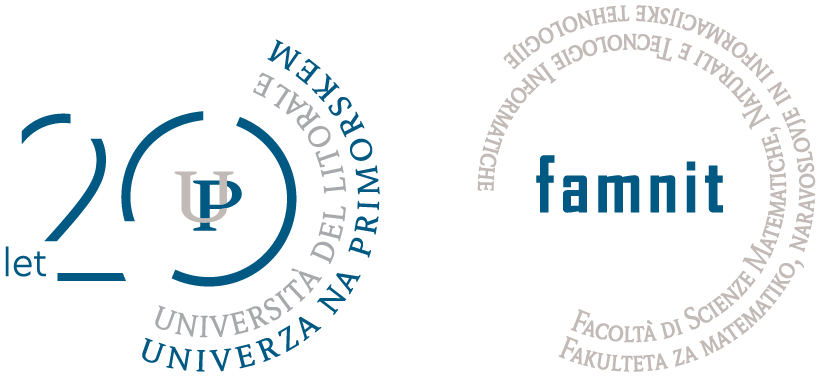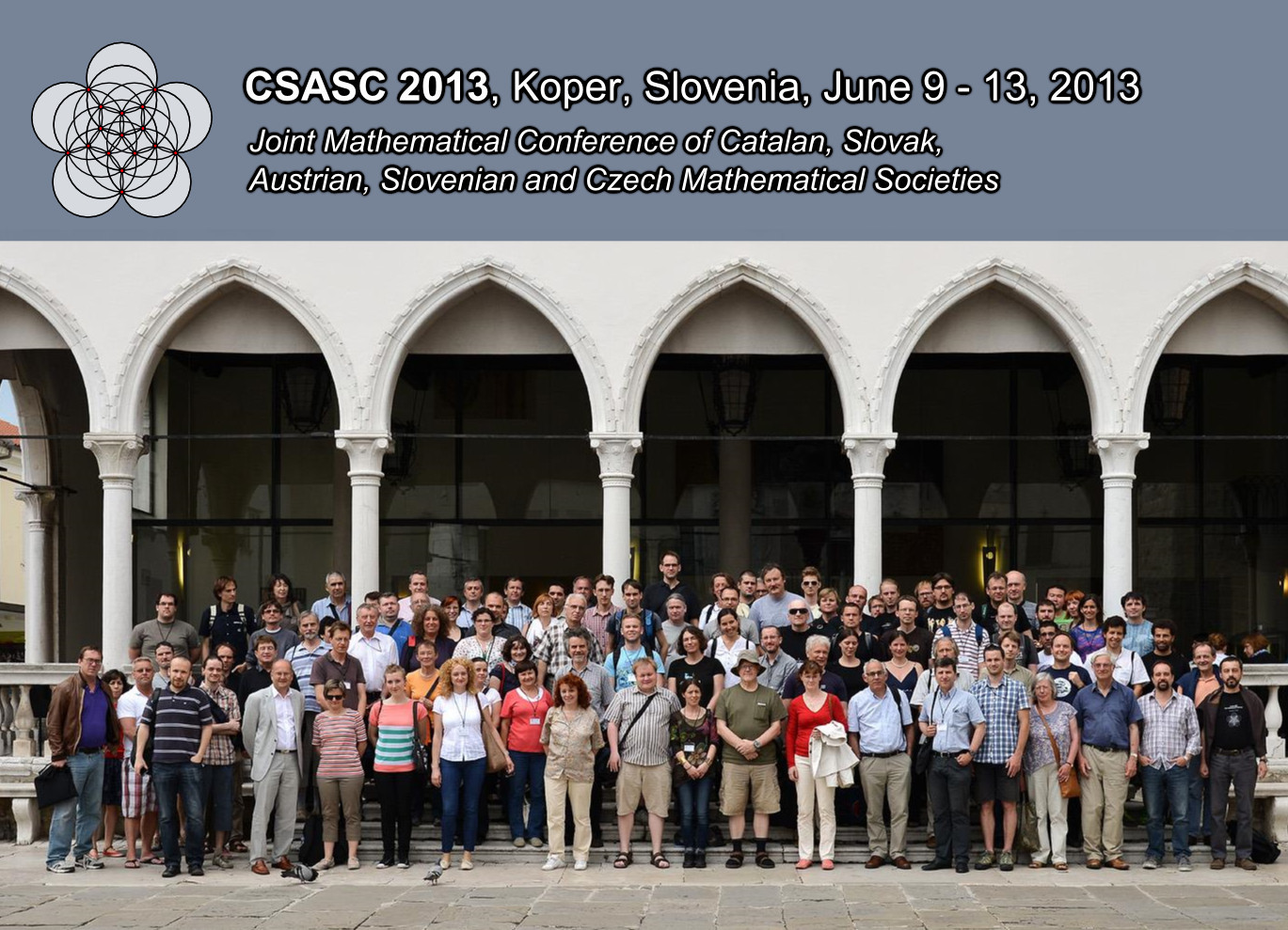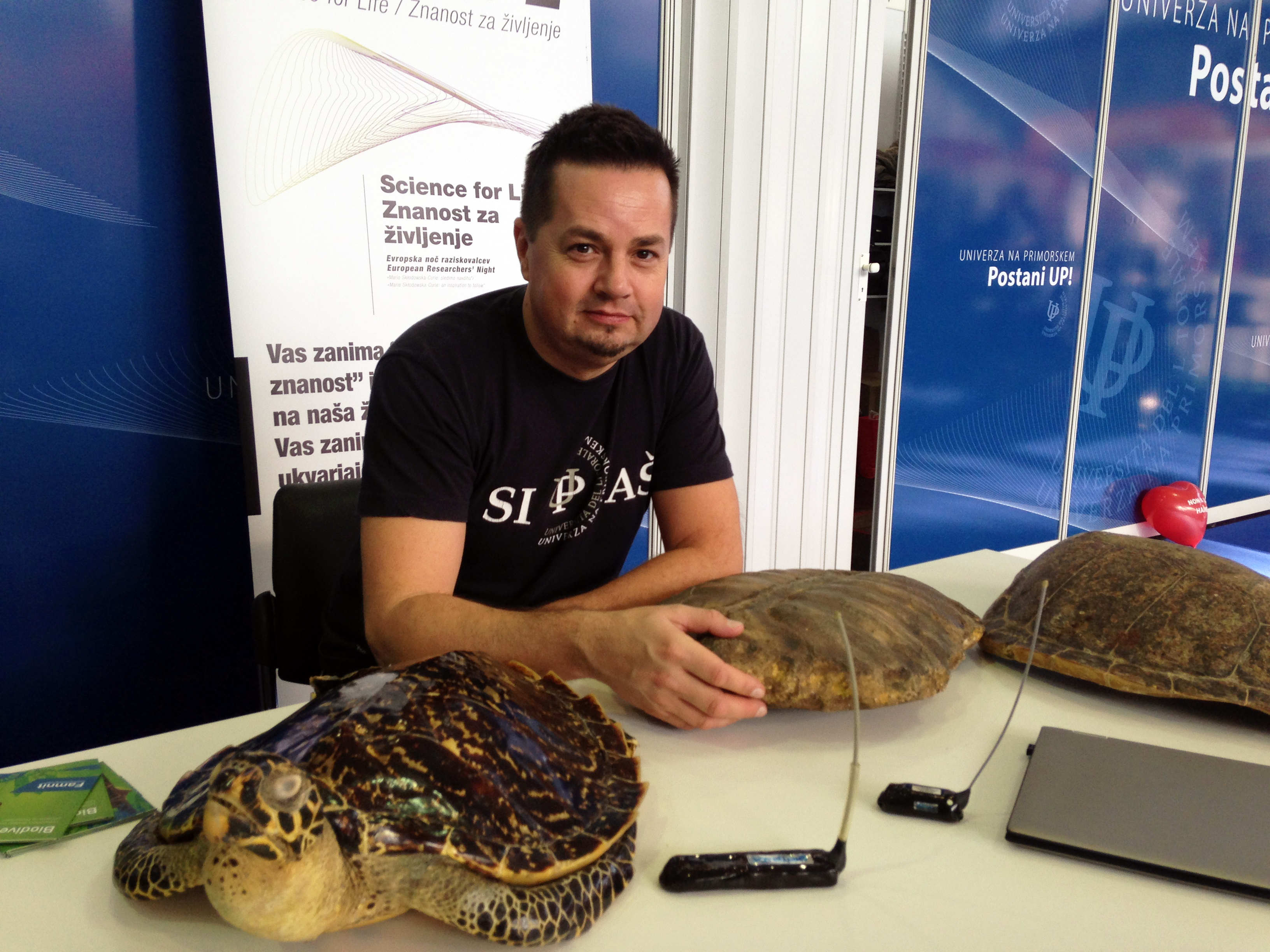University of Primorska Faculty of Mathematics, Natural Sciences and Information TechnologiesSI | EN# Mathematical Research Seminar

Mathematical research seminar is organized by the departments of Mathematics of two members of the University - UP FAMNIT and Andrej Marušič Institute (UP IAM), every Monday from October to June.

You are cordially invited to attend the lectures.

Archive
Datum in ura / Date and time: 3.4.23
(15:00 -- 16:00)
Predavalnica / Location: FAMNIT-MP1
Predavatelj / Lecturer: Igor Klep (University of Ljubljana, Slovenia)
Naslov / Title: Similarity of matrix tuples
Vsebina / Abstract:

Two matrices A,B are called similar if there is an invertible matrix P satisfying AP=PB. As is well known, complex matrices are up to similarity uniquely determined by their Jordan canonical form. This talk will discuss possible extensions to (joint) similarity of tuples of matrices. Tuples (A_1,...,A_n) and (B_1,...,B_n) are called similar if there is an invertible matrix P such that A_jP=PB_j for all j. The classification of matrix tuples up to similarity has been deemed a “hopeless problem”, but is widely studied due to its importance in multiple areas of mathematics, ranging from operator theory, invariant and representation theory and algebraic geometry to algebraic statistics and computational complexity. In this talk we shall present a new natural collection of separating invariants for matrix tuples, along the way solving a 2003 conjecture of Hadwin and Larson.

This is based on joint work with Harm Derksen, Visu Makam and Jurij Volčič.

We look forward to sharing the passion for math with you!